Processing ......FreeComputerBooks.com Links to Free Computer, Mathematics, Technical Books all over the World

Uncertainty in Engineering: Introduction to Methods and Applications
🌠 Top Free Computer Networking Books - 100% Free or Open Source!
• Title: Uncertainty in Engineering: Introduction to Methods and Applications
• Author(s) Louis J. M. Aslett, Frank P. A. Coolen, Jasper De Bock
• Publisher: Springer (December 9, 2021); eBook (Creative Commons Licensed)
• Paperback: 156 pages
• eBook: PDF and ePub
• Language: English
• ASIN: B09ND2V6ZG
• ISBN-10: 3030836398
• ISBN-13: 978-3030836399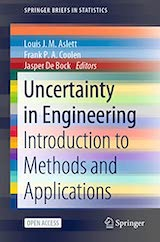Book Description

This open access book provides an introduction to uncertainty quantiﬁcation in engineering. Starting with preliminaries on Bayesian statistics and Monte Carlo methods, followed by material on imprecise probabilities, it then focuses on reliability theory and simulation methods for complex systems.

The ﬁnal two chapters discuss various aspects of Aerospace Engineering, considering stochastic model updating from an imprecise Bayesian perspective, and uncertainty quantiﬁcation for aerospace ﬂight modelling.

• Louis Aslett is an Associate Professor in the Department of Mathematical Sciences at Durham University.
• Frank Coolen is a Professor in the Department of Mathematical Sciences at Durham University.
• Jasper De Bock is an Assistant Professor in the Department of Electronics and Information Systems at Ghent University.
Reviews, Ratings, and Recommendations: Related Book Categories: Read and Download Links:Similar Books:
•An Introduction to Bayesian Thinking (Merlise Clyde, et al.)

It provides an introduction to Bayesian Inference in decision making without requiring calculus. It may be used on its own as an open-access introduction to Bayesian inference using R Programming for anyone interested in learning about Bayesian statistics.

•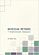Bayesian Methods for Statistical Analysis (Borek Puza)

Bayesian methods for statistical analysis is a book on statistical methods for analysing a wide variety of data. It contains many exercises, all with worked solutions, including complete computer code.

•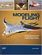Modeling Flight (Joseph R. Chambers)

It describes the issues that must be considered when transferring subscale results to full-scale application, focuses on the applications of the techniques to generic configurations for general research as well as to specific aircraft and spacecraft configurations.

•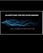Algorithms for Decision Making (Mykel Kochenderfer, et al)

This textbook provides a broad introduction to algorithms for decision making under uncertainty, covering the underlying mathematical problem formulations and the algorithms for solving them.

•Control Theory Tutorial: Illustrated by Software Examples

This open access book introduces the basic principles of control theory in a concise self-study guide. It complements the classic texts by emphasizing the simple conceptual unity of the subject. A novice can quickly see how and why the different parts fit together.

•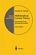Mathematical Control Theory (Eduardo D. Sontag)

This book covers what constitutes the common core of control theory and is unique in its emphasis on foundational aspects. While covering a wide range of topics written in a standard theorem/proof style, it also develops the necessary techniques from scratch.

•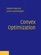Convex Optimization (Stephen Boyd, et al.)

On recognizing convex optimization problems and then finding the most appropriate technique for solving them. It contains many worked examples in fields such as engineering, computer science, mathematics, statistics, finance, and economics.

•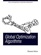Global Optimization Algorithms - Theory and Application

This book is devoted to global optimization algorithms, which are methods to find optimal solutions for given problems. It especially focuses on Evolutionary Computation by discussing evolutionary algorithms, genetic algorithms, Genetic Programming, etc.

•Feedback Control Theory (John C. Doyle, et al)

An excellent introduction to feedback control system design, this book offers a theoretical approach that captures the essential issues and can be applied to a wide range of practical problems.

•Feedback Systems: An Introduction for Scientists and Engineers

This book provides an introduction to the mathematics needed to model, analyze, and design feedback systems. It uses techniques from physics, computer science, and operations research to introduce control-oriented modeling.

•Control Systems (WikiBooks)

This book will discuss the topic of Control Systems, which is an interdisciplinary engineering topic. Methods considered here will consist of both "Classical" control methods, and "Modern" control methods.

•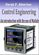Control Engineering: An Introduction with the Use of MATLAB

The book covers the basic aspects of linear single loop feedback control theory. Explanations of the mathematical concepts used in classical control such as root loci, frequency response and stability methods are explained by making use of MATLAB.

Book Categories
 :All CategoriesTop Free BooksRecent BooksMiscellaneous BooksComputer EngineeringComputer LanguagesComputer ScienceData Science/DatabasesJava and Java EE (J2EE)Linux and UnixMathematicsMicrosoft and .NETMobile ComputingNetworking and CommunicationsSoftware EngineeringSpecial TopicsWeb Programming
Other Categories